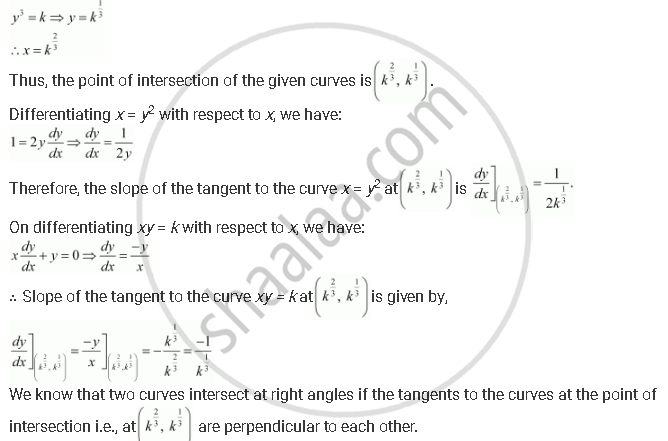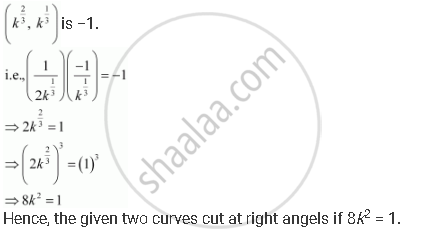Share

# Prove that the Curves X = Y2 and Xy = K Cut at Right Angles If 8k2 = 1. [Hint: Two Curves Intersect at Right Angle If the Tangents to the Curves at the Point of Intersection Are Perpendicular to Each Other.] - CBSE (Commerce) Class 12 - Mathematics

#### Question

Prove that the curves x = y2 and xy = k cut at right angles if 8k2 = 1. [Hint: Two curves intersect at right angle if the tangents to the curves at the point of intersection are perpendicular to each other.]

#### Solution

The equations of the given curves are given as x =  y^2 and xy = k

Putting x = y2 in xy = k, we get:This implies that we should have the product of the tangents as − 1.

Thus, the given two curves cut at right angles if the product of the slopes of their respective tangents atIs there an error in this question or solution?

#### APPEARS IN

NCERT Solution for Mathematics Textbook for Class 12 (2018 to Current)
Chapter 6: Application of Derivatives
Q: 23 | Page no. 213

#### Video TutorialsVIEW ALL 

Solution Prove that the Curves X = Y2 and Xy = K Cut at Right Angles If 8k2 = 1. [Hint: Two Curves Intersect at Right Angle If the Tangents to the Curves at the Point of Intersection Are Perpendicular to Each Other.] Concept: Tangents and Normals.
S